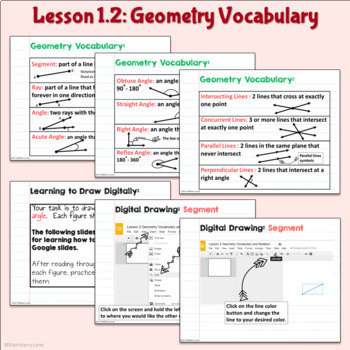# Geometry Vocabulary Tools Intro to Proofs Digital Lessons BundleSubject
Resource Type
File Type

Zip

(10 MB|220 pages)
Standards
6 Products in this Bundle
6 products
• Bundle Description
• StandardsNEW
Going Digital this year? Not ready to give up all paper and pencil activities?

This is an introductory unit to Geometry. It is a paperless digital lessons blended with paper and pencil labs. Lesson format is compatible with Google slides®
This unit focuses on geometry vocabulary terms: the undefined terms, types of angles and line. An exploratory lesson allows students to discover the linear pair theorem and the vertical angles theorem. The unit continues with how to use geometric tools, followed by a review of how to solve equations for algebra and ending with solving beginner level proofs. An editable unit assessment has 2 levels for differentiation and a rubric for grading.

Lesson 1: Undefined Terms
Lesson 2: Geometry Vocabulary
Lesson 3 Lab*: Using Geometric Tools
Lesson 4: Angle Pair Relationships
Lesson 5: Algebra and Angles
Differentiated Unit Assessment with grading rubric

Do you have independent learners or students who like to self check their work?
There is a Grade as you Go option to let students self-assess as they complete the lesson.

#TPTtech
Make formal geometric constructions with a variety of tools and methods (compass and straightedge, string, reflective devices, paper folding, dynamic geometric software, etc.).
Prove theorems about lines and angles.
Know precise definitions of angle, circle, perpendicular line, parallel line, and line segment, based on the undefined notions of point, line, distance along a line, and distance around a circular arc.
Explain each step in solving a simple equation as following from the equality of numbers asserted at the previous step, starting from the assumption that the original equation has a solution. Construct a viable argument to justify a solution method.
Use facts about supplementary, complementary, vertical, and adjacent angles in a multi-step problem to write and solve simple equations for an unknown angle in a figure.
Total Pages
220 pages
N/A
Teaching Duration
1 Week
Report this Resource to TpT
Reported resources will be reviewed by our team. Report this resource to let us know if this resource violates TpT’s content guidelines.\$27.72
Bundle
List Price:
\$34.65
You Save:
\$6.93